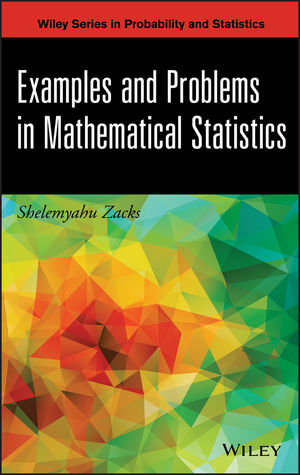## minimaxity of a Bayes estimator

Posted in Books, Kids, Statistics, University life with tags , , , , , on February 2, 2015 by xi'anToday, while in Warwick, I spotted on Cross Validated a question involving “minimax” in the title and hence could not help but look at it! The way I first understood the question (and immediately replied to it) was to check whether or not the standard Normal average—reduced to the single Normal observation by sufficiency considerations—is a minimax estimator of the normal mean under an interval zero-one loss defined by$\mathcal{L}(\mu,\hat{\mu})=\mathbb{I}_{|\mu-\hat\mu|>L}=\begin{cases}1 &\text{if }|\mu-\hat\mu|>L\\ 0&\text{if }|\mu-\hat{\mu}|\le L\\ \end{cases}$

where L is a positive tolerance bound. I had not seen this problem before, even though it sounds quite standard. In this setting, the identity estimator, i.e., the normal observation x, is indeed minimax as (a) it is a generalised Bayes estimator—Bayes estimators under this loss are given by the centre of an equal posterior interval—for this loss function under the constant prior and (b) it can be shown to be a limit of proper Bayes estimators and its Bayes risk is also the limit of the corresponding Bayes risks. (This is a most traditional way of establishing minimaxity for a generalised Bayes estimator.) However, this was not the question asked on the forum, as the book by Zacks it referred to stated that the standard Normal average maximised the minimal coverage, which amounts to the maximal risk under the above loss. With the strange inversion of parameter and estimator in the minimax risk:$\sup_\mu\inf_{\hat\mu} R(\mu,\hat{\mu})\text{ instead of } \sup_\mu\inf_{\hat\mu} R(\mu,\hat{\mu})$

which makes the first bound equal to 0 by equating estimator and mean μ. Note however that I cannot access the whole book and hence may miss some restriction or other subtlety that would explain for this unusual definition. (As an aside, note that Cross Validated has a protection against serial upvoting, So voting up or down at once a large chunk of my answers on that site does not impact my “reputation”!)